# 高维空间中具有间接信号产出生物趋化模型解的全局有界性Global Boundedness of Classical Solutions to a High-Dimensional Chemotaxis Model with Indirect Signal Production

DOI: 10.12677/AAM.2019.84088, PDF, HTML, XML, 下载: 258  浏览: 380  科研立项经费支持

Abstract: This paper deals with the chemotaxis system of diffusion and aggregation of Mountain Pine Beetle with indirect attractant production and generalized logistic source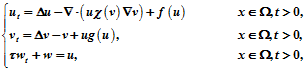in a smoothly bounded domain with homoge-neous Neumann boundary conditions and nonnegative initial values, the chemotactic sensitivity function satisfies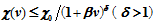the consumption function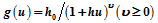and the logistic source satisfiesMoreover,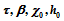are given positive parameter. Firstly, the energy estimation method and Gagliardo-Nirenberg inequality are used to establish the local prior estimate of u and w, and then Moser iteration is used. This problem admits a unique global classical solution that is uniformly in-time bounded.

1. 引言

$\left\{\begin{array}{l}{u}_{t}=\Delta u-\chi \nabla \cdot \left(u\nabla v\right),\text{\hspace{0.17em}}\text{\hspace{0.17em}}\text{\hspace{0.17em}}\text{\hspace{0.17em}}\text{\hspace{0.17em}}\text{\hspace{0.17em}}\text{\hspace{0.17em}}\text{\hspace{0.17em}}\text{\hspace{0.17em}}\text{\hspace{0.17em}}\text{\hspace{0.17em}}\text{\hspace{0.17em}}\text{\hspace{0.17em}}\text{\hspace{0.17em}}\text{\hspace{0.17em}}\text{\hspace{0.17em}}\text{\hspace{0.17em}}\text{ }\text{\hspace{0.17em}}\text{\hspace{0.17em}}x\in \Omega ,\text{\hspace{0.17em}}t>0,\\ {v}_{t}=\Delta v-v+u,\text{\hspace{0.17em}}\text{\hspace{0.17em}}\text{\hspace{0.17em}}\text{\hspace{0.17em}}\text{\hspace{0.17em}}\text{\hspace{0.17em}}\text{\hspace{0.17em}}\text{\hspace{0.17em}}\text{\hspace{0.17em}}\text{\hspace{0.17em}}\text{\hspace{0.17em}}\text{\hspace{0.17em}}\text{\hspace{0.17em}}\text{\hspace{0.17em}}\text{\hspace{0.17em}}\text{\hspace{0.17em}}\text{\hspace{0.17em}}\text{\hspace{0.17em}}\text{\hspace{0.17em}}\text{\hspace{0.17em}}\text{\hspace{0.17em}}\text{\hspace{0.17em}}\text{\hspace{0.17em}}\text{\hspace{0.17em}}\text{\hspace{0.17em}}\text{\hspace{0.17em}}\text{\hspace{0.17em}}\text{\hspace{0.17em}}x\in \Omega ,\text{\hspace{0.17em}}t>0,\end{array}$ (1.1)

$\left\{\begin{array}{l}{u}_{t}=\Delta u-\nabla \cdot \left(u\chi \left(v\right)\nabla v\right)+f\left(u\right)\text{\hspace{0.17em}}\text{\hspace{0.17em}}\text{\hspace{0.17em}}\text{\hspace{0.17em}}\text{\hspace{0.17em}}\text{\hspace{0.17em}}\text{\hspace{0.17em}}\text{ }\text{ }\text{ }\text{ }\text{ }\text{\hspace{0.17em}}\text{\hspace{0.17em}}\text{\hspace{0.17em}}\text{\hspace{0.17em}}\text{\hspace{0.17em}}\text{\hspace{0.17em}}\text{\hspace{0.17em}}\text{\hspace{0.17em}}\text{\hspace{0.17em}}\text{\hspace{0.17em}}\text{\hspace{0.17em}}\text{\hspace{0.17em}}\text{\hspace{0.17em}}\text{\hspace{0.17em}}\text{ }\text{ }\text{ }\text{ }\text{\hspace{0.17em}}\text{ }\text{ }x\in \Omega ,t>0,\\ {v}_{t}=\Delta v-v+ug\left(u\right),\text{ }\text{ }\text{ }\text{ }\text{ }\text{ }\text{ }\text{ }\text{ }\text{ }\text{ }\text{ }\text{ }\text{ }\text{ }\text{ }\text{ }\text{ }\text{ }\text{ }\text{ }\text{ }\text{ }\text{ }\text{ }\text{ }\text{ }\text{ }\text{ }\text{ }\text{ }\text{ }\text{ }\text{ }\text{ }\text{ }\text{ }\text{ }\text{ }\text{ }\text{ }\text{ }\text{ }\text{ }\text{ }\text{ }\text{ }\text{ }\text{\hspace{0.17em}}\text{\hspace{0.17em}}\text{\hspace{0.17em}}\text{\hspace{0.17em}}\text{\hspace{0.17em}}\text{\hspace{0.17em}}\text{\hspace{0.17em}}\text{\hspace{0.17em}}\text{\hspace{0.17em}}\text{\hspace{0.17em}}\text{\hspace{0.17em}}\text{\hspace{0.17em}}\text{\hspace{0.17em}}\text{\hspace{0.17em}}\text{\hspace{0.17em}}\text{\hspace{0.17em}}\text{\hspace{0.17em}}\text{\hspace{0.17em}}\text{\hspace{0.17em}}\text{\hspace{0.17em}}\text{\hspace{0.17em}}\text{\hspace{0.17em}}\text{\hspace{0.17em}}\text{\hspace{0.17em}}\text{ }\text{\hspace{0.17em}}\text{ }x\in \Omega ,t>0,\\ \tau {w}_{t}+w=u,\text{ }\text{ }\text{ }\text{ }\text{ }\text{ }\text{ }\text{ }\text{ }\text{ }\text{ }\text{ }\text{ }\text{ }\text{ }\text{ }\text{ }\text{ }\text{ }\text{ }\text{ }\text{ }\text{ }\text{ }\text{ }\text{ }\text{ }\text{ }\text{ }\text{ }\text{ }\text{ }\text{ }\text{ }\text{ }\text{ }\text{ }\text{ }\text{ }\text{ }\text{ }\text{ }\text{ }\text{ }\text{ }\text{ }\text{ }\text{ }\text{ }\text{ }\text{ }\text{ }\text{ }\text{ }\text{ }\text{ }\text{ }\text{ }\text{ }\text{ }\text{ }\text{ }\text{ }\text{ }\text{\hspace{0.17em}}\text{\hspace{0.17em}}\text{\hspace{0.17em}}\text{\hspace{0.17em}}\text{\hspace{0.17em}}\text{\hspace{0.17em}}\text{\hspace{0.17em}}\text{\hspace{0.17em}}\text{\hspace{0.17em}}\text{\hspace{0.17em}}\text{\hspace{0.17em}}\text{\hspace{0.17em}}\text{\hspace{0.17em}}\text{\hspace{0.17em}}\text{\hspace{0.17em}}\text{\hspace{0.17em}}\text{\hspace{0.17em}}\text{\hspace{0.17em}}\text{\hspace{0.17em}}\text{\hspace{0.17em}}\text{\hspace{0.17em}}\text{\hspace{0.17em}}\text{\hspace{0.17em}}\text{\hspace{0.17em}}\text{\hspace{0.17em}}\text{\hspace{0.17em}}\text{\hspace{0.17em}}\text{ }\text{ }\text{\hspace{0.17em}}\text{\hspace{0.17em}}\text{\hspace{0.17em}}x\in \Omega ,t>0,\\ \frac{\partial u}{\partial \nu }=\frac{\partial v}{\partial \nu }=\frac{\partial w}{\partial \nu }=0,\text{\hspace{0.17em}}\text{\hspace{0.17em}}\text{\hspace{0.17em}}\text{\hspace{0.17em}}\text{\hspace{0.17em}}\text{\hspace{0.17em}}\text{\hspace{0.17em}}\text{\hspace{0.17em}}\text{\hspace{0.17em}}\text{\hspace{0.17em}}\text{\hspace{0.17em}}\text{\hspace{0.17em}}\text{\hspace{0.17em}}\text{\hspace{0.17em}}\text{\hspace{0.17em}}\text{\hspace{0.17em}}\text{\hspace{0.17em}}\text{\hspace{0.17em}}\text{\hspace{0.17em}}\text{\hspace{0.17em}}\text{\hspace{0.17em}}\text{\hspace{0.17em}}\text{\hspace{0.17em}}\text{\hspace{0.17em}}\text{\hspace{0.17em}}\text{\hspace{0.17em}}\text{\hspace{0.17em}}\text{\hspace{0.17em}}\text{\hspace{0.17em}}\text{\hspace{0.17em}}\text{\hspace{0.17em}}\text{\hspace{0.17em}}\text{\hspace{0.17em}}\text{\hspace{0.17em}}\text{\hspace{0.17em}}\text{\hspace{0.17em}}\text{\hspace{0.17em}}\text{\hspace{0.17em}}\text{\hspace{0.17em}}\text{\hspace{0.17em}}\text{\hspace{0.17em}}\text{ }\text{}x\in \partial \Omega ,\text{\hspace{0.17em}}t>0,\\ u\left(x,0\right)={u}_{0}\left(x\right),\text{\hspace{0.17em}}v\left(x,0\right)={v}_{0}\left(x\right),\text{\hspace{0.17em}}w\left(x,0\right)={w}_{0}\left(x\right),\text{\hspace{0.17em}}\text{}x\in \Omega ,\end{array}$ (1.2)

$\chi \left(v\right)\le {\chi }_{0}/{\left(1+\beta v\right)}^{\delta },\text{\hspace{0.17em}}\delta >1,\text{\hspace{0.17em}}{\chi }_{0}>0,$ (1.3)

$f\left(u\right)\le au-b{u}^{2},\text{\hspace{0.17em}}a,b>0,\text{\hspace{0.17em}}f\left(0\right)=0,$ (1.4)

$g\left(u\right)={h}_{0}/{\left(1+hu\right)}^{\upsilon },\text{\hspace{0.17em}}{h}_{0},h,\upsilon \ge 0.$ (1.5)

2. 预备知识

$\begin{array}{l}u\in {C}^{0}\left(\stackrel{¯}{\Omega }×\left[0,{T}_{\mathrm{max}}\right)\right)\cap {C}^{2,1}\left(\stackrel{¯}{\Omega }×\left[0,{T}_{\mathrm{max}}\right)\right),\\ v\in {C}^{0}\left(\stackrel{¯}{\Omega }×\left[0,{T}_{\mathrm{max}}\right)\right)\cap {L}_{loc}^{\infty }\left(\left[0,{T}_{\mathrm{max}}\right),{W}^{1,q}\left(\Omega \right)\right)\cap {C}^{2,1}\left(\stackrel{¯}{\Omega }×\left[0,{T}_{\mathrm{max}}\right)\right),\\ w\in {C}^{0}\left(\stackrel{¯}{\Omega }×\left[0,{T}_{\mathrm{max}}\right)\right)\cap {C}^{2,1}\left(\stackrel{¯}{\Omega }×\left[0,{T}_{\mathrm{max}}\right)\right),\end{array}$

${‖u\left(x,t\right)‖}_{{L}^{\infty }\left(\Omega \right)}+{‖v\left(x,t\right)‖}_{{w}^{1,q}\left(\Omega \right)}+{‖w\left(x,t\right)‖}_{{L}^{\infty }\left(\Omega \right)}\to \infty ,t\to {T}_{\mathrm{max}}.$

${\int }_{\Omega }u\left(\cdot ,t\right)\text{d}x\le {M}_{0},\text{\hspace{0.17em}}t\in \left(0,{T}_{\mathrm{max}}\right),$ (2.1)

${\int }_{\Omega }w\left(\cdot ,t\right)\text{d}x\le {M}_{1},t\in \left(0,{T}_{\mathrm{max}}\right),$ (2.3)

$\frac{\text{d}}{\text{d}t}{\int }_{\Omega }\tau w\text{d}x+{\int }_{\Omega }w\text{d}x={\int }_{\Omega }u\text{d}x,$

${\int }_{\Omega }w\left(\cdot ,t\right)\text{d}x\le {M}_{1},\text{\hspace{0.17em}}t\in \left(0,{T}_{\mathrm{max}}\right).$

${‖\psi ‖}_{{L}^{\alpha }\left(\Omega \right)}\le {C}_{GN}\left({‖\nabla \psi ‖}_{{L}^{2}\left(\Omega \right)}^{{\lambda }^{\ast }}{‖\psi ‖}_{{L}^{h}\left(\Omega \right)}^{1-{\lambda }^{\ast }}+{‖\psi ‖}_{{L}^{h}\left(\Omega \right)}\right),$

${\lambda }^{\ast }=\frac{\frac{n}{h}-\frac{n}{\alpha }}{1-\frac{n}{2}+\frac{n}{h}}.$

3. 解的全局有界性

${‖u\left(\cdot ,t\right)‖}_{{L}^{k}\left(\Omega \right)}\le {M}_{2},\text{\hspace{0.17em}}t\in \left(0,{T}_{\mathrm{max}}\right),$ (3.1)

${‖w\left(\cdot ,t\right)‖}_{{L}^{k}\left(\Omega \right)}\le {M}_{3},\text{\hspace{0.17em}}t\in \left(0,{T}_{\mathrm{max}}\right),$ (3.2)

$\varphi \left(s\right)=\mathrm{exp}\left({\left(1+{\beta }^{\ast }s\right)}^{-r}\right),\text{\hspace{0.17em}}s>0.$

$k>1$$r>1$

$r\le \mathrm{min}\left\{k-1/8k,2\delta -2\right\},$ (3.3)

$r\le k\left(\frac{k-1}{e{C}_{GN}^{2}{k}^{2}{M}^{k\left(1-\lambda \right)}}-a\right),$ (3.4)

${\beta }^{\ast }>{\chi }_{0}\sqrt{2p\left(p-1\right)/r\left(r+1\right)},$ (3.5)$\begin{array}{l}=-\left(k-1\right){\int }_{\Omega }{u}^{k-2}\varphi \left(v\right){|\nabla u|}^{2}\text{d}x-{\int }_{\Omega }{u}^{k-1}{\varphi }^{\prime }\left(v\right)\nabla u\cdot \nabla v\text{d}x\\ \text{\hspace{0.17em}}\text{\hspace{0.17em}}+\left(k-1\right){\int }_{\Omega }{u}^{k-1}\varphi \left(v\right)\chi \left(v\right)\nabla u\cdot \nabla v\text{d}x+{\int }_{\Omega }{u}^{p}{\varphi }^{\prime }\left(v\right)\chi \left(v\right){|\nabla v|}^{2}\text{d}x\\ \text{\hspace{0.17em}}\text{\hspace{0.17em}}+\frac{1}{k}{\int }_{\Omega }{u}^{k}\varphi \left(v\right)f\left(u\right)\text{d}x-{\int }_{\Omega }{u}^{k-1}{\varphi }^{\prime }\left(v\right)\nabla u\cdot \nabla v\text{d}x-\frac{1}{k}{\int }_{\Omega }{u}^{k}{\varphi }^{″}\left(v\right){|\nabla v|}^{2}\text{d}x\\ \text{\hspace{0.17em}}\text{\hspace{0.17em}}-\frac{1}{k}{\int }_{\Omega }{u}^{k}v{\varphi }^{\prime }\left(v\right)\text{d}x+\frac{1}{k}{\int }_{\Omega }{u}^{k}{\varphi }^{\prime }\left(v\right)g\left(u\right)\text{d}x\end{array}$

$\begin{array}{l}\frac{1}{k}\frac{\text{d}}{\text{d}t}{\int }_{\Omega }{u}^{k}\varphi \left(v\right)\text{d}x+\left(k-1\right){\int }_{\Omega }{u}^{k-2}\varphi \left(v\right){|\nabla u|}^{2}\text{d}x+\frac{1}{k}{\int }_{\Omega }{u}^{k}{\varphi }^{″}\left(v\right){|\nabla v|}^{2}\text{d}x\\ \le -2{\int }_{\Omega }{u}^{k-1}{\varphi }^{\prime }\left(v\right)\nabla u\cdot \nabla vdx+\left(k-1\right){\int }_{\Omega }{u}^{k-1}\varphi \left(v\right)\chi \left(v\right)\nabla u\cdot \nabla v\text{d}x\\ \text{\hspace{0.17em}}\text{\hspace{0.17em}}-\frac{1}{k}{\int }_{\Omega }{u}^{k}v{\varphi }^{\prime }\left(v\right)\text{d}x+a{\int }_{\Omega }{u}^{k}\varphi \left(v\right)\text{d}x,\end{array}$ (3.6)

$-\frac{1}{k}{\int }_{\Omega }{u}^{k}v{\varphi }^{\prime }\left(v\right)\text{d}x\le \frac{r}{k}{\int }_{\Omega }{u}^{k}\varphi \left(v\right)\text{d}x,$

$-2{\int }_{\Omega }{u}^{k-1}{\varphi }^{\prime }\left(v\right)\nabla u\cdot \nabla v\text{d}x\le \frac{k-1}{4}{\int }_{\Omega }{u}^{k-2}\varphi \left(v\right){|\nabla u|}^{2}\text{d}x+\frac{4}{k-1}{\int }_{\Omega }{u}^{k}\frac{{{\varphi }^{\prime }}^{2}\left(v\right)}{\varphi \left(v\right)}{|\nabla v|}^{2}\text{d}x,$

$\begin{array}{l}\left(k-1\right){\int }_{\Omega }{u}^{k-1}\varphi \left(v\right)\chi \left(v\right)\nabla u\cdot \nabla v\text{d}x\\ \le \frac{k-1}{4}{\int }_{\Omega }{u}^{k-2}\varphi \left(v\right){|\nabla u|}^{2}\text{d}x+\left(k-1\right){\int }_{\Omega }{u}^{k}\varphi \left(v\right){\chi }^{2}\left(v\right){|\nabla v|}^{2}\text{d}x\\ \le \frac{k-1}{4}{\int }_{\Omega }{u}^{k-2}\varphi \left(v\right){|\nabla u|}^{2}\text{d}x+\left(k-1\right){\chi }_{0}^{2}{\int }_{\Omega }{u}^{k}{\left(1+\beta v\right)}^{-2\delta }\varphi \left(v\right){|\nabla v|}^{2}\text{d}x,\end{array}$

$\begin{array}{l}\frac{1}{k}\frac{\text{d}}{\text{d}t}{\int }_{\Omega }{u}^{k}\varphi \left(v\right)\text{d}x+\frac{k-1}{2}{\int }_{\Omega }{u}^{k-2}\varphi \left(v\right){|\nabla u|}^{2}\text{d}x+\frac{1}{k}{\int }_{\Omega }{u}^{k}{\varphi }^{″}\left(v\right){|\nabla v|}^{2}\text{d}x\\ \le \frac{4}{k-1}{\int }_{\Omega }{u}^{k}\frac{{{\varphi }^{\prime }}^{2}\left(v\right)}{\varphi \left(v\right)}{|\nabla v|}^{2}\text{d}x+\left(k-1\right){\chi }_{0}^{2}{\int }_{\Omega }{u}^{k}{\left(1+\beta v\right)}^{-2\delta }\varphi \left(v\right){|\nabla v|}^{2}\text{d}x\\ \text{\hspace{0.17em}}\text{\hspace{0.17em}}\text{ }+\left(\frac{r}{k}+a\right){\int }_{\Omega }{u}^{p}\varphi \left(v\right)\text{d}x,\end{array}$ (3.7)

${Z}_{1}\left(s\right):=\frac{4}{k-1}\frac{{{\varphi }^{\prime }}^{2}\left(s\right)}{\varphi \left(s\right)}=\frac{4}{k-1}\cdot {\left({\beta }^{\ast }\right)}^{2}{r}^{2}{\left(1+{\beta }^{\ast }s\right)}^{-2r-2}{\text{e}}^{{\left(1+{\beta }^{\ast }s\right)}^{-r}},$

${Z}_{2}\left(s\right):=\left(k-1\right){\chi }_{0}^{2}{\left(1+\beta s\right)}^{-2\delta }\varphi \left(s\right)=\left(k-1\right){\chi }_{0}^{2}{\left(1+\beta s\right)}^{-2\delta }{\text{e}}^{{\left(1+{\beta }^{\ast }s\right)}^{-r}}$

${Z}_{3}\left(s\right):=\frac{1}{k}{\varphi }^{″}\left(s\right)=\frac{1}{k}\cdot {\left({\beta }^{\ast }\right)}^{2}r\left(r+1\right){\left(1+{\beta }^{\ast }s\right)}^{-r-2}{\text{e}}^{{\left(1+{\beta }^{\ast }s\right)}^{-r}}+\frac{1}{k}\cdot {\left({\beta }^{\ast }\right)}^{2}{r}^{2}{\left(1+{\beta }^{\ast }s\right)}^{-2r-2}{\text{e}}^{{\left(1+{\beta }^{\ast }s\right)}^{-r}},$

$\begin{array}{c}\frac{{Z}_{1}\left(s\right)}{\frac{1}{2}{Z}_{3}\left(s\right)}\le \frac{\frac{4}{k-1}\cdot {\left({\beta }^{\ast }\right)}^{2}{r}^{2}{\left(1+{\beta }^{\ast }s\right)}^{-2r-2}{\text{e}}^{{\left(1+{\beta }^{\ast }s\right)}^{-r}}}{\frac{1}{2}\cdot \frac{1}{k}\cdot {\left({\beta }^{\ast }\right)}^{2}r\left(r+1\right){\left(1+{\beta }^{\ast }s\right)}^{-r-2}{\text{e}}^{{\left(1+{\beta }^{\ast }s\right)}^{-r}}}\\ =\frac{8kr}{\left(k-1\right)\left(r-1\right)}\cdot {\left(1+{\beta }^{\ast }s\right)}^{-r}\le \frac{8kr}{k-1}\le 1,\end{array}$

$\begin{array}{c}\frac{{Z}_{2}\left(s\right)}{\frac{1}{2}{Z}_{3}\left(s\right)}\le \frac{\left(k-1\right){\chi }_{0}^{2}{\left(1+\beta s\right)}^{-2\delta }{\text{e}}^{{\left(1+{\beta }^{\ast }s\right)}^{-r}}}{\frac{1}{2}\cdot \frac{1}{k}\cdot {\left({\beta }^{\ast }\right)}^{2}r\left(r+1\right){\left(1+{\beta }^{\ast }s\right)}^{-r-2}{\text{e}}^{{\left(1+{\beta }^{\ast }s\right)}^{-r}}}\\ =\frac{2k\left(k-1\right){\chi }_{0}^{2}}{{\left({\beta }^{\ast }\right)}^{2}r\left(r+1\right)}\cdot {\left(1+\beta s\right)}^{-2\delta }{\left(1+{\beta }^{\ast }s\right)}^{r+2},\end{array}$

$\begin{array}{c}{\phi }^{\prime }\left(s\right)={\left(1+\beta s\right)}^{-2\delta -1}\cdot {\left(1+{\beta }^{\ast }s\right)}^{r+1}\cdot \left\{-2k\beta \left(1+{\beta }^{\ast }s\right)+\left(r+2\right)\left(1+\beta s\right)\right\}\\ \le {\left(1+\beta s\right)}^{-2\delta -1}\cdot {\left(1+{\beta }^{\ast }s\right)}^{r+1}\cdot \left\{-\left(2\delta \beta -r-2\right)-\left[2\delta \beta {\beta }^{\ast }-\left(r+2\right)\beta \right]s\right\}\\ \le 0,\text{\hspace{0.17em}}s\ge 0,\end{array}$

$\frac{{Z}_{2}\left(s\right)}{\frac{1}{2}{Z}_{3}\left(s\right)}\le \frac{2k\left(k-1\right){\chi }_{0}^{2}}{{\left({\beta }^{\ast }\right)}^{2}r\left(r+1\right)}\le 1,\text{\hspace{0.17em}}s\ge 0,$

$\frac{1}{k}\frac{\text{d}}{\text{d}t}{\int }_{\Omega }{u}^{k}\varphi \left(v\right)\text{d}x+\frac{k-1}{2}{\int }_{\Omega }{u}^{k-2}\varphi \left(v\right){|\nabla u|}^{2}\text{d}x\le \left(a+\frac{r}{k}\right){\int }_{\Omega }{u}^{k}\varphi \left(v\right)\text{d}x，\text{\hspace{0.17em}}t\in \left(0,{T}_{\mathrm{max}}\right),$

$\begin{array}{c}{\int }_{\Omega }{u}^{k}\varphi \left(v\right)\text{d}x\le e{\int }_{\Omega }{u}^{k}\text{d}x=e{‖{u}^{\frac{k}{2}}‖}_{{L}^{2}\left(\Omega \right)}^{2}\le e\cdot {C}_{GN}{‖{u}^{\frac{k}{2}}‖}_{{W}^{1，2}\left(\Omega \right)}^{2h}\cdot {‖{u}^{\frac{k}{2}}‖}_{{L}^{\frac{2}{k}}\left(\Omega \right)}^{2\left(1-h\right)}\\ \le e\cdot {c}_{GN}\cdot {\left(c\left(\frac{2}{k}\right)\right)}^{2h}{\left({‖\nabla {u}^{\frac{k}{2}}‖}_{{L}^{2}\left(\Omega \right)}+{‖{u}^{\frac{k}{2}}‖}_{{L}^{\frac{2}{k}}\left(\Omega \right)}\right)}^{2h}\cdot {‖{u}^{\frac{k}{2}}‖}_{{L}^{\frac{2}{k}}\left(\Omega \right)}^{2\left(1-h\right)},\end{array}$

$h=\frac{\frac{2n}{k}-\frac{n}{2}}{\frac{2n}{k}+1-\frac{n}{2}}\in \left(0,1\right),$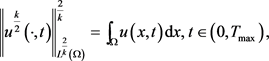${\int }_{\Omega }{u}^{k}\varphi \left(v\right)\text{d}x\le {c}_{1}{\left({‖\nabla {u}^{\frac{k}{2}}‖}^{2}{}_{{L}^{2}\left(\Omega \right)}+1\right)}^{h},\text{\hspace{0.17em}}{c}_{1}>0,$

$\begin{array}{c}\frac{k-1}{2}{\int }_{\Omega }{u}^{k-2}\varphi \left(v\right){|\nabla u|}^{2}\text{d}x\ge \frac{k-1}{2}{\int }_{\Omega }{u}^{k-2}{|\nabla u|}^{2}\text{d}x=\frac{2\left(k-1\right)}{{k}^{2}}{\int }_{\Omega }{|\nabla {u}^{\frac{k}{2}}|}^{2}\text{d}x\\ \ge \frac{2\left(k-1\right)}{{k}^{2}{c}_{1}{}^{\frac{1}{h}}}{\left({\int }_{\Omega }{u}^{k}\varphi \left(v\right)\text{d}x\right)}^{\frac{1}{h}}-\frac{2\left(k-1\right)}{{k}^{2}},\end{array}$

$\begin{array}{l}\frac{1}{k}\frac{\text{d}}{\text{d}t}{\int }_{\Omega }{u}^{k}\varphi \left(v\right)\text{d}x\\ \le -\frac{2\left(k-1\right)}{{k}^{2}{c}_{1}{}^{\frac{1}{h}}}{\left({\int }_{\Omega }{u}^{k}\varphi \left(v\right)\text{d}x\right)}^{\frac{1}{h}}+\frac{2\left(k-1\right)}{{k}^{2}}+\left(a+\frac{r}{k}\right){\int }_{\Omega }{u}^{k}\varphi \left(v\right)\text{d}x,\text{\hspace{0.17em}}t\in \left(0,{T}_{\mathrm{max}}\right),\end{array}$

${‖u\left(\cdot ,t\right)‖}_{{L}^{k}\left(\Omega \right)}\le {M}_{2},\text{\hspace{0.17em}}t\in \left(0,{T}_{\mathrm{max}}\right),$

$\frac{\tau }{p}\frac{\text{d}}{\text{d}t}{\int }_{\Omega }{w}^{k}\text{d}x+{\int }_{\Omega }{w}^{k}\text{d}x={\int }_{\Omega }u{w}^{k-1}\text{d}x,$

${\int }_{\Omega }u{w}^{k-1}\text{d}x\le \frac{1}{4}{\int }_{\Omega }{w}^{k}\text{d}x+{C}_{k}{\int }_{\Omega }{u}^{k}\text{d}x,\text{\hspace{0.17em}}{C}_{k}>0,$

$\frac{\tau }{k}\frac{\text{d}}{\text{d}t}{\int }_{\Omega }{w}^{k}\text{d}x+\frac{3}{4}{\int }_{\Omega }{w}^{k}\text{d}x\le {M}_{3},$

${‖w\left(\cdot ,t\right)‖}_{{L}^{k}\left(\Omega \right)}\le {M}_{3},\text{\hspace{0.17em}}t\in \left(0,{T}_{\mathrm{max}}\right),$

4. 定理1的证明

${‖u\left(x,t\right)‖}_{{L}^{\infty }\left(\Omega \right)}+{‖v\left(x,t\right)‖}_{{L}^{\infty }\left(\Omega \right)}+{‖w\left(x,t\right)‖}_{{L}^{\infty }\left(\Omega \right)}\le c\left(\tau \right),\text{\hspace{0.17em}}t\in \left(\tau ,{T}_{\mathrm{max}}\right),$

$v\left(\cdot ,t\right)={\text{e}}^{t\left(\Delta -1\right)}{v}_{0}+{\int }_{0}^{t}{\text{e}}^{\left(t-s\right)\left(\Delta -1\right)}g\left(u\left(\cdot ,t\right)\right)\text{d}s,\text{\hspace{0.17em}}t\in \left(0,{T}_{\mathrm{max}}\right),$

$\begin{array}{c}{‖v\left(\cdot ,t\right)‖}_{{W}^{1,2p}\left(\Omega \right)}\le c{‖{\left(\Delta +1\right)}^{\rho }v\left(\cdot ,t\right)‖}_{{L}^{q}\left(\Omega \right)}\\ \le c{t}^{-\rho }{\text{e}}^{-\upsilon t}{‖{v}_{0}‖}_{{L}^{q}\left(\Omega \right)}+c{\int }_{0}^{t}{\left(t-s\right)}^{-\rho }{\text{e}}^{-\upsilon \left(t-s\right)}{‖u\left(\cdot ,t\right)‖}_{{L}^{q}\left(\Omega \right)}{‖g\left(u\left(\cdot ,t\right)\right)‖}_{{L}^{q}\left(\Omega \right)}\text{d}s\\ \le c{t}^{-\rho }+c{\int }_{0}^{t}{\left(t-s\right)}^{-\rho }{\text{e}}^{-\upsilon \left(t-s\right)}\text{d}s\le c{t}^{-\rho }+c{\int }_{0}^{\infty }{\sigma }^{-\rho }{\text{e}}^{-\upsilon \sigma }\text{d}\sigma \\ \le c\left({t}^{-\rho }+1\right),\text{\hspace{0.17em}}t\in \left(0,{T}_{\mathrm{max}}\right),\end{array}$

${‖v\left(\cdot ,t\right)‖}_{{L}^{\infty }\left(\Omega \right)}\le {M}_{4},\text{\hspace{0.17em}}t\in \left(0,{T}_{\mathrm{max}}\right).$

$\begin{array}{c}\frac{\text{d}}{\text{d}t}{\int }_{\Omega }{u}^{m}\text{d}x=m{\int }_{\Omega }{u}^{m-1}{u}_{t}\text{d}x\\ =m{\int }_{\Omega }{u}^{m-1}\Delta u\text{d}x-m{\int }_{\Omega }{u}^{m-1}\nabla \cdot \left(u\chi \left(v\right)\nabla v\right)\text{d}x+m{\int }_{\Omega }{u}^{m-1}f\left(u\right)\text{d}x\\ =-m\left(m-1\right){\int }_{\Omega }{u}^{m-2}{|\nabla u|}^{2}\text{d}x+m{\int }_{\Omega }{u}^{m-1}f\left(u\right)\text{d}x+m\left(m-1\right){\int }_{\Omega }{u}^{m-1}\chi \left(v\right)\nabla u\cdot \nabla v\text{d}x\\ \le \frac{-4\left(m-1\right)}{m}{\int }_{\Omega }{|\nabla {u}^{\frac{m}{2}}|}^{2}\text{d}x+cm\left(m-1\right){\int }_{\Omega }{u}^{m-1}|\nabla u|\text{d}x+am{\int }_{\Omega }{u}^{m}\text{d}x\\ \le \frac{-2\left(m-1\right)}{m}{\int }_{\Omega }{|\nabla {u}^{\frac{m}{2}}|}^{2}\text{d}x+\left(\frac{{c}^{2}m\left(m-1\right)}{2}+am\right){\int }_{\Omega }{u}^{m}\text{d}x,\end{array}$

$\frac{\text{d}}{\text{d}t}{\int }_{\Omega }{u}^{m}\text{d}x+m\left(m-1\right){\int }_{\Omega }{u}^{m}\text{d}x\le \frac{-2\left(m-1\right)}{m}{\int }_{\Omega }{|\nabla {u}^{\frac{m}{2}}|}^{2}\text{d}x+{c}_{1}m\left(m-1\right){\int }_{\Omega }{u}^{m}\text{d}x,\text{\hspace{0.17em}}{c}_{1}>0,$

${c}_{1}m\left(m-1\right){\int }_{\Omega }{u}^{m}\text{d}x\le \frac{2\left(m-1\right)}{m}{\int }_{\Omega }{|\nabla {u}^{\frac{m}{2}}|}^{2}\text{d}x+{c}_{2}m\left(m-1\right){\left({\int }_{\Omega }{u}^{\frac{m}{2}}\text{d}x\right)}^{2},\text{\hspace{0.17em}}{c}_{2}>0,$

$\frac{\text{d}}{\text{d}t}{\int }_{\Omega }{u}^{m}\text{d}x+m\left(m-1\right){\int }_{\Omega }{u}^{m}dx\le {c}_{2}m\left(m-1\right){\left({\int }_{\Omega }{u}^{\frac{m}{2}}\text{d}x\right)}^{2},\text{\hspace{0.17em}}{c}_{2}>0,$

${\int }_{\Omega }{u}^{m}\text{d}x\le {\int }_{\Omega }{u}_{0}^{m}\text{d}x+{c}_{2}\underset{0\le t\le {T}_{\mathrm{max}}}{\mathrm{sup}}{\left({\int }_{\Omega }{u}^{\frac{m}{2}}\text{d}x\right)}^{2},\text{\hspace{0.17em}}{c}_{2}>0,$

$G\left(m\right)=\mathrm{max}\left\{{‖{u}_{0}‖}_{{L}^{\infty }\left(\Omega \right)},\underset{0\le t\le {T}_{\mathrm{max}}}{\mathrm{sup}}{\left({\int }_{\Omega }{u}^{m}\text{d}x\right)}^{\frac{1}{m}}\right\}\le {c}_{3}^{\frac{1}{m}}G\left(m/2\right),$

$m={2}^{i},\text{\hspace{0.17em}}i=1,2,\cdots$ ，那么可知

$G\left({2}^{i}\right)\le {c}_{3}^{{2}^{-i}}G\left({2}^{i-1}\right)\le {c}_{3}^{{2}^{-i}+{2}^{-i+1}}G\left({2}^{i-2}\right)\le \cdots \le {c}_{3}G\left(1\right),$

$i\to \infty$ ，可以得到

${‖u\left(\cdot ,t\right)‖}_{{L}^{\infty }\left(\Omega \right)}\le {M}_{5},\text{\hspace{0.17em}}t\in \left(0,{T}_{\mathrm{max}}\right).$

$\begin{array}{c}w\left(x,t\right)=\frac{1}{\tau }{\int }_{0}^{t}{\text{e}}^{\frac{1}{\tau }\left(s-t\right)}u\left(x,s\right)\text{d}s+{w}_{0}{\text{e}}^{-\frac{1}{\tau }t}\\ \le \frac{1}{\tau }{‖{\int }_{0}^{t}{\text{e}}^{\frac{1}{\tau }\left(s-t\right)}u\left(x,s\right)\text{d}s‖}_{{L}^{\infty }\left(\Omega \right)}+{‖{w}_{0}{\text{e}}^{-\frac{1}{\tau }t}‖}_{{L}^{\infty }\left(\Omega \right)}\\ \le {C}_{1}\cdot \tau \left(1-{\text{e}}^{-\frac{1}{\tau }t}\right)+{C}_{2}{\text{e}}^{-\frac{1}{\tau }t}\\ \le {C}_{1}\cdot \tau +{C}_{2}:={M}_{6},t\in \left(0,{T}_{\mathrm{max}}\right),\end{array}$

${‖w\left(x,t\right)‖}_{{L}^{\infty }\left(\Omega \right)}\le {M}_{6},t\in \left(0,{T}_{\mathrm{max}}\right).$

${‖u\left(x,t\right)‖}_{{L}^{\infty }\left(\Omega \right)}+{‖v\left(x,t\right)‖}_{{L}^{\infty }\left(\Omega \right)}+{‖w\left(x,t\right)‖}_{{L}^{\infty }\left(\Omega \right)}\le C,\text{\hspace{0.17em}}t\in \left(\tau ,{T}_{\mathrm{max}}\right).$

5. 结论

NOTES

*通讯作者。

  Keller, E.F. and Sege, L.A. (1970) Initiation of Slime Mold Aggregation Viewed as an Instability. Journal of Theoretical Biology, 26, 399-415. https://doi.org/10.1016/0022-5193(70)90092-5  Osaki, K. and Yagi, A. (2001) Finite Dimensional Attractors for One-Dimensional Keller-Segel Equations. Funkcialaj Ekvacioj, 44, 441-469.  Nagai, T., Senba, T. and Yoshida, K. (1997) Application of the Trudinger-Moser Inequality to a Parabolic System of Chemotaxis. Funkcialaj Ekvacioj, 40, 411-433.  Horstmann, D. and Wang, G. (2001) Blow-Up in a Chemotaxis Model without Symmetry Assumptions. European Journal of Applied Mathematics, 12, 159-177. https://doi.org/10.1017/S0956792501004363  Nagai, T. (2001) Blow up of Nonradial Solutions to Parabol-ic-Elliptic Systems Modeling Chemotaxis in Two-Dimensional Domains. Journal of Inequalities and Applications, 6, 37-55. https://doi.org/10.1155/S1025583401000042  Tao, Y.S. and Winkler, M. (2012) Boundedness in a Quasilinear Parabolic-Parabolic Keller-Segel System with Subcritical Sensitivity. Journal of Differential Equations, 252, 692-715. https://doi.org/10.1155/S1025583401000042  Winkler, M. (2013) Finite-Time Blow-Up in the Higher-Dimensional Parabolic-Parabolic Keller-Segel System. Journal de Mathématiques Pures et Appliquées, 100, 748-767. https://doi.org/10.1016/j.matpur.2013.01.020  Winkler, M. (2010) Absence of Collapse in a Parabolic Chemotaxis System with Signal-Dependent Sensitivity. Mathematische Narchrichten, 283, 1664-1673.  Hu, B. and Tao, Y.S. (2016) To the Exclusion of Blow-Up in a Three-Dimensional Chemotaxis-Growth Model with Indirect Attractant Production. Mathematical Models and Methods in Applied Sciences, 26, 2111-2128. https://doi.org/10.1142/S0218202516400091  王笑丹, 陶有山. 具间接信号产出的二维趋向性模型古典解的整体存在性和有界性[J]. 东华大学学报(自然科学版), 2016, 42(6): 922-930.  Li, H.Y. and Tao, Y.S. (2018) Boundedness in a Chemotaxis System with Indirect Signal Production and Generalized Logistic Source. Applied Mathematics Letters, 77, 108-113. https://doi.org/10.1016/j.aml.2017.10.006  Li, Y. (2015) Global Bounded Solutions and Their Asymptotic Properties under Small Initial Data Condition in a Two-Dimensional Chemotax Is System for Two Species. Journal of Mathematical Analysis and Applications, 429, 1291-1304. https://doi.org/10.1016/j.jmaa.2015.04.052  Cho, Y.S. and Wang, Z.A. (2010) Prevention of Blow-Up by Fast Diffusion in Chemotaxis. Journal of Mathematical Analysis and Applications, 362, 553-564. https://doi.org/10.1016/j.jmaa.2009.08.012  Delgado, M., Gayte, I., Morales-rodrigo, C. and Suarez, A. (2010) An Angiogenesis Model with Nonlinear Chemotactic Response and Flux at the Tumor Boundary. Nonlinear Analysis: Theory, Methods & Applications, 72, 330-347. https://doi.org/10.1016/j.na.2009.06.057